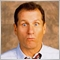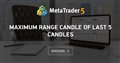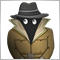# Trying to find the biggest body of the last X candles49

Hello, I am trying to find the biggest body of the last X (in this case 3 ) candles. Any help is greatly appreciated.

This is what i have so far:

```   int index=0;
double delta=0;
double delta2=0;

for(int i=0;i<3;i++)
{
// if (delta<High[i]-Low[i])
if (delta<Open[i]-Close[i] || delta2<Close[i]-Open[i])
{
delta=Open[i]-Close[i];
delta2=Close[i]-Open[i];
index=i;
}
}```

Picked up the original piece of code from here: https://www.mql5.com/en/forum/192383#comment_5025296Maximum range candle of last 5 candles
• 2017.05.11
• www.mql5.com
Hello, I want to identify the candle (in the last 5) that have maximum range (High[i]-Low[i]...7896

```   int    index = 0;
double delta = 0;
for ( int i=0; i< 3; i++)
{
if (delta < MathAbs(Open[i]-Close[i]) )
{
delta = MathAbs(Open[i]-Close[i]);
index = i;
}
}```49

Awesome, works perfectly.

Many thanks!